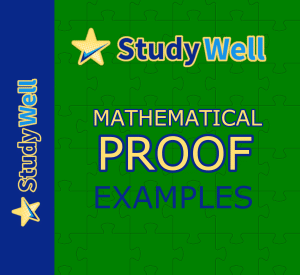# Proof – mathematical logic and reasoning

## PROOF

According to the Collins Dictionary, ‘proof is a fact, argument, or piece of evidence which shows that something is definitely true or definitely exists’. In Mathematics, we use logical processes and fundamental tools to show that mathematical statements are true. We call this Mathematical Proof

There are numerous proof methods in mathematics. Typically, you will study Proof by Deduction and Exhaustion  in the first year of A-Level Maths or equivalent. Students will also be taught Disproof by CounterExample to show them how to prove that a mathematical statement isn’t true. You don’t usually see Proof by Contradiction until the second year.